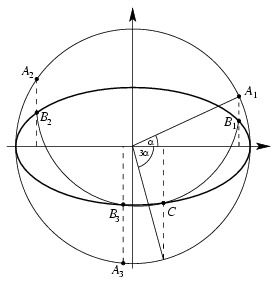Mathematical and Physical Journal
for High Schools
Issued by the MATFUND Foundation
 Already signed up? New to KöMaL?# Solutions for advanced problems "A" in March, 2002

In this page only the sketch of the solutions are published; in some cases only the final results. To achieve the maximum score in the competition more detailed solutions needed.

A. 287. Someone has drawn an ellipse on a sheet of paper, and he has also marked the endpoints of its axes. The major axis is twice as long as the minor axis. Find a construction method for dividing any given acute angle into three equal parts, using a pair of compasses, a straight edge, and the given ellipse only.

Solution. Let 3$\displaystyle alpha$ be the angle to be trisected, and let the equation of the ellipse in rectangular coordinates be x2+4y2=1.Let Ai=(cos($\displaystyle alpha$+(i-1).120o);sin($\displaystyle alpha$+(i-1).120o)) and $\displaystyle B_i=(\cos(\alpha+(i-1)\cdot120^\circ);{1\over2}\sin(\alpha+(i-1)\cdot120^\circ))$ (i=1,2,3). The points Ai are on the unit circle, and the points Bi are on the ellipse. The task is to construct these points.

Consider the circle passing through the points Bi. The circle also intersects the ellipse at a fourth point C. With a little calculation, it can be checked that the centre of the circle is $\displaystyle K=\left({3\over16}\cos3\alpha;{3\over8}\sin3\alpha\right)$ and that $\displaystyle C=(\cos3\alpha;{1\over2}\sin3\alpha)$. For a given 3$\displaystyle alpha$, the circle can be constructed, and it intersects the ellipse at the points Bi.

A. 288. Let p be an n degree polynomial, where n$\displaystyle ge$1. Prove that there exist at least n+1 complex numbers yielding 0 or 1 as the value of f. (IMC 7, London, 2000)

Solution. Let z1, ..., zk be the complex numbers where the value of p is 0 or 1. Let $\displaystyle mu$j denote the multiplicity of the root zj of the polynomial p(z) or p(z)-1. Then the number zj is a root of p' with a multiplicity of ($\displaystyle mu$j-1). If multiplicity counts, The polynomials p(z) and p(z)-1 together have 2n roots among the numbers z1,...,zk, and the polynomial p' has at most n-1, thus

$\displaystyle mu$1+...+$\displaystyle mu$k=2n,   ($\displaystyle mu$1-1)+...+($\displaystyle mu$k-1)$\displaystyle le$n-1.

By subtraction, we get k$\displaystyle ge$n+1.

A. 289. Prove that if the function f is defined on the set of positive real numbers, its values are real, and f satisfies the equation

$\displaystyle f\left({x+y\over2}\right)+f\left({2xy\over x+y}\right)=f(x)+f(y)$

for all positive x,y, then

$\displaystyle 2f\big(\sqrt{xy}\big)=f(x)+f(y)$

for every positive number pair xy. (Miklós Schweitzer Memorial Competition, 2001)

Solution. Let a,b,c,d be positive real numbers. By applying the functional equation several times, we have

f(a)+f(b)+f(c)+f(d)=

$\displaystyle =f\left({a+b\over2}\right)+f\left({2ab\over a+b}\right)+f\left({c+d\over2}\right)+f\left({2cd\over c+d}\right)=$

$\displaystyle =f\left({{a+b\over2}+{c+d\over2}\over2}\right)+f\left({2{a+b\over2}\cdot{c+d\over2}\over{a+b\over2}+{c+d\over2}}\right)+$

$\displaystyle \qquad+f\left({{2ab\over a+b}+{2cd\over c+d}\over2}\right)+f\left({2{2ab\over a+b}\cdot{2cd\over c+d}\over{2ab\over a+b}+{2cd\over c+d}}\right)=$

$\displaystyle f\left({a+b+c+d\over4}\right)+f\left({(a+b)(c+d)\over a+b+c+d}\right)+$

$\displaystyle \qquad+f\left({abc+abd+acd+bcd\over(a+b)(c+d)}\right)+f\left({4abcd\over abc+abd+acd+bcd}\right).$

By repeating the above procedure with b and c interchanged, we get

 (1) $\displaystyle f\left({(a+b)(c+d)\over a+b+c+d}\right)+f\left({abc+abd+acd+bcd\over(a+b)(c+d)}\right)=$

$\displaystyle \qquad=f\left({(a+c)(b+d)\over a+b+c+d}\right)+f\left({abc+abd+acd+bcd\over(a+c)(b+d)}\right).$

Let a=c, b=a2/d and $\displaystyle t={a\over b}+{b\over a}$. It is easy to check that

$\displaystyle {(a+b)(c+d)\over a+b+c+d}={abc+abd+acd+bcd\over(a+b)(c+d)}=a,$

$\displaystyle {(a+c)(b+d)\over a+b+c+d}=a\cdot{2t\over2+t},$

and finally

$\displaystyle {abc+abd+acd+bcd\over(a+c)(b+d)}=a\cdot{2+t\over2t}.$

Substitution of the results into (1) gives

 (2) $\displaystyle 2f(a)=f\left(a\cdot{2t\over2+t}\right)+f\left(a\cdot{2+t\over2t}\right)$

t can be any number not smaller than 2, and $\displaystyle {2t\over2+t}$ can be any number not smaller than 1. Thus for every number pair x$\displaystyle ge$y there exist the numbers a and t, such that $\displaystyle a\cdot{2t\over2+t}=x$, $\displaystyle a\cdot{2+t\over2t}=y$ and $\displaystyle \sqrt{xy}=a$.